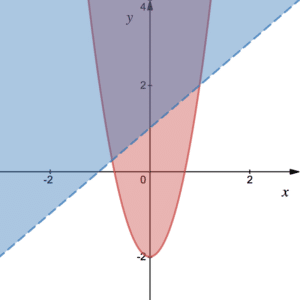# Inequalities

## Things you need to know about Inequalities

• If the question has inequalities,  the solution must include inequalities. If your solution has an equals sign then you have gone wrong.
• We solve linear inequalities in very much the same way as linear equations. See Example 1.
• We solve quadratic inequalities by first finding the roots of the quadratic. See more on Quadratics. Use the graph to identify where the quadratic is positive or negative. If the graph has two separate regions then the answer must have two separate regions. See Example 2.
• You can plot inequalities on a number line. So, if you want to see where two hold simultaneously look for where they overlap. See Example 3. Set notation may also be given or required in some questions. See Example 2. For instance, $x< a$ OR $x> b$  is equivalent to $\left\lbrace x:x< a\right\rbrace\cup \left\lbrace x:x>b\right\rbrace$. Similarly,  $x< c$ AND $x> d$  is equivalent to $\left\lbrace x:x< c\right\rbrace\cap \left\lbrace x:x>d\right\rbrace$. See Venn Diagrams for more information on union and intersection.

Who invented the inequality symbols and when?

## Representing Inequalities GraphicallyWe can represent Inequalities  graphically. Consider $y> x+1$ and $y\geq 4x^2-2$ and imagine them on a graph. The blue region represents the former. The red region represents the latter. Notice the use of the dashed line to show inequality while the solid lines shows equality. When the red solid line is above the blue dashed line the following inequality holds: $4x^2-2> x+1$. Use standard techniques to find where the curves intersect to identify the appropriate regions.

## When the Inequality is reversed

The following points are very important facts about inequalities in addition to the above bullet points:

1. Multiplying or dividing both sides by a negative number reverses the sign of the inequality. To solve $-ax> b$ where $a$ is positive, add $ax$ to both sides to get $0> b+ax$. Then, subtract $b$ from both sides to get $-b> ax$ or $-\frac{b}{a}> x$ since a is positive. Written as $x< -\frac{b}{a}$ we can think of the sign as being reversed.
2. Taking reciprocals of both sides also reverses the inequality. In order to solve $\frac{a}{x}< b$ we must multiply both sides by $x^2$. This is because we don’t know the sign of $x$ but we do know $x^2$ is positive. It follows that $ax< bx^2$ – we don’t reverse the sign of the inequality. Finally, we have $ax-bx^2=x(a-bx)< 0$ which can be solved as any other quadratic inequality (see above). Be careful to take note of the signs of a and b.

See Example 4.

## Examples of Indices

Solve the inequality $2x+3\hspace{2pt}\geq \hspace{2pt}5(x-2)+1$.

Solve the following: $x^2-x\hspace{2pt}>\hspace{2pt} 6$.

Find the region where $2x+3\hspace{2pt}\geq \hspace{2pt}5x-9$ AND $x^2-x\hspace{2pt}>\hspace{2pt} 6$.

Solve the following inequalities:

1. $-x\leq 4$
2. $\frac{1}{x}\hspace{2pt}>\hspace{2pt}7$